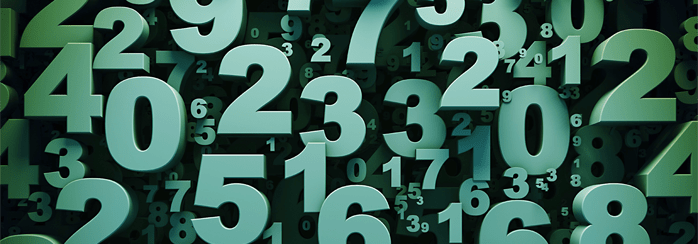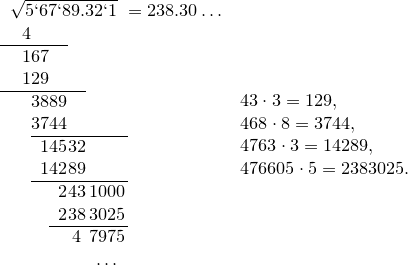# Finding Square Root Without a CalculatorAll of us widely use calculators to perform mathematical operations. Obviously, these machines were invented by people, who determined their work according to the certain algorithms for quick count. In this article, we’re going to tell you about one of such algorithms. We’ll also show how you can extract square roots in column and you’ll see that the result of this operation can be received with arbitrary precision; we can find any digits number in its decimal representation, even if it turns irrational.So, let’s take a look at how the algorithm works on this example:

Take an arbitrary number…$56789,321$

1. Divide its numbers into pairs: those, which are lying on the left of the decimal point, form the groups by two from the right to the left, and those which are on the right form pairs from left to right. So, we get the following  $5 67 89,32` 1$.

2. Extract the square root of the first digit group on the left. In this case, we have number $5$ (it is clear that the exact root cannot be removed, take the number whose square is the closest to our number, but does not exceed it). Here, it is the number $2$. Write $2$ in response as a significant digit of the root.

3. We raise to the square the already received number (which is $2$) and subtract it from the first left digit group (number $5$). In this case, $1$ remains.

4. Place the next set of two digits (that is $67$) to the right side and get the result – $167$.

5. Now, be attentive. We need to ascribe a number $b$ to the number $4$ on the right, thus the multiplication of the number $\overline {4b}$  by $b$ gives us the result as close as possible to $167$, but not more. In our case, it is number $3$. It should be written in the answer next to the $2$, on the right.

6. Subtract the product of $43 \cdot 3 = 129$ from the $167$ and get $38$.

7. Repeat the same operation: ascribe next group of numbers ($89$) to $38$ on the right, and  multiply $23$ by $2$. We ascribe one digit to the resulting number $46$ on the right, so that the result of multiplication by the number would be less than $3889$, but the closest to it. In the given example, $8$ is the next number in a decimal notation root.

8. Next, we meet the decimal point in the number and we should put the same in the result, after the number $8$. Continue the process, move down two digits after the decimal point. You can move down like this even two zeros.
All described calculations can be written as follows:This algorithm is based on the formula

$(10a+b)^2=100a^2+20ab+b^2=100a^2+(20a+b)b$

First, we subtract the square of number, attributing one digit to the result; at the same time, we ascribe two decimal digits under the radical. Hence, we use splitting into pairs (seen from the formula). Subtracting the square of number, you should subtract the number in the form of$(20a+b)b$. It’s very simple and there isn’t any magic.

Read our blog, follow us on YouTube and G+ to learn more interesting tricks in math and other subjects.

4 Shares
Filed under Math.